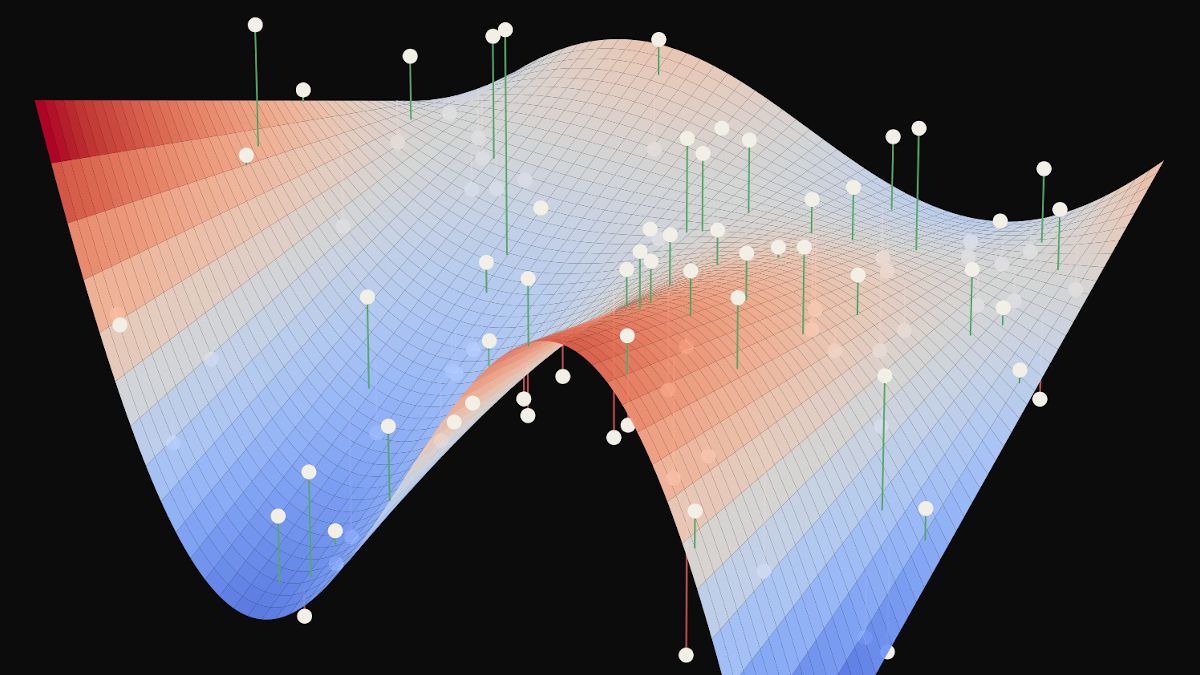# Uncovering the limits of model discoveryMachine learning is making front pages. Whether it is a new algorithm that draws watercolors from any imaginable prompt, or one that chats with humans with encyclopedic knowledge and polite manners, the number of tasks that computers can solve as well as humans (or even better) seems to be growing quickly. Much of this progress is due to deep learning, which involves building huge mathematical models with millions or billions of parameters. Because of the sheer size of these models, however, when an algorithm malfunctions or misbehaves, it is often impossible to pinpoint the reason. In fact, even when they work as expected, it is hard to really understand why.

In science and engineering, such models are sometimes not good enough. For example, when scientists get data from an experiment in the lab, they are often less interested in huge models that accurately reproduce their results, than in simple closed-form mathematical models that strike a balance between accuracy and interpretability. After all, this is how much of our knowledge of the world has been acquired over the last centuries: observing phenomena and building simple closed-form mathematical models for them, which hopefully can be linked to plausible mechanisms. Think, for instance, of Kepler's laws and Newton's law of universal gravitation, and how they allowed us to understand important aspects of the physical world, well beyond the motion of planets.

The area of machine learning that aims at discovering simple closed-form mathematical models from data is variously known, in different disciplines, as symbolic regression, equation discovery or systems identification. Here, the goal is to build "machine scientists" that are able to take data as input and build simple mathematical models, similar to Kepler's or Newton's laws.

But is it always possible to do so? Even when there is a simple model that describes the data perfectly, except for observational inaccuracies and noise, can we always discover it? So far, the implicit assumption in the field has been that we can. In a new paper in Nature Communications, we have now shown that, indeed, sometimes we can —but sometimes we cannot.

When the observational noise is low, the true model can always be discovered, provided that one deals properly with the uncertainty associated to each possible model. This is quite remarkable, considering that there is a virtually infinite number of possible models; the key is to use a rigorous (so-called Bayesian) approach that deals precisely with probabilities. This approach is connected to the information-theoretical idea that the best model is the one that best summarizes (or compresses, as in a ZIP file) the data. And, since it is the best summary of the data, it also makes the best predictions about unseen data —in the low-noise regime, even deep neural networks make less accurate extrapolations than machine scientists.

However, when observational noise grows, there is a point at which a large amount of models become as plausible as the true model. Beyond this point, not even the true model is able to summarize the data better than many others because much of the data is actually noise. Therefore, in this regime, no machine scientist, ever, will be able to uncover the model that truly generated the data. Importantly, the transition between the low-noise (discoverable or learnable) and the high-noise (undiscoverable or unlearnable) regimes happens abruptly, in which seems to be a phase transition.

The study helps to uncover what seems to be a fundamental limitation of machine science. More broadly, it opens the door to new theoretical studies on the limits of machine learning.